# Geometry

## Objective

Identify and determine values of angles in complementary and supplementary relationships.

## Common Core Standards

### Core Standards

?

• 7.G.B.5 — Use facts about supplementary, complementary, vertical, and adjacent angles in a multi-step problem to write and solve simple equations for an unknown angle in a figure.

?

• 4.MD.C.5

• 4.MD.C.7

## Criteria for Success

?

1. Understand that complementary angles are angles whose measures add up to 90°, and supplementary angles are angles whose measures add up to 180°.
2. Identify pairs of complementary and supplementary angles in angle diagrams.
3. Find the values of angles using complementary and supplementary angle relationships and equations.

## Tips for Teachers

?

Students studied angles in fourth grade, where they recognized angles as shapes formed when two rays share a common endpoint. They understood that angle measures are additive, and they solved addition and subtraction problems to find missing angles. In this lesson, students formally define complementary and supplementary angles, and they start to develop their understanding of angle relationships and how they can represent these relationships using equations.

#### Fishtank Plus

• Problem Set
• Student Handout Editor
• Vocabulary Package

## Anchor Problems

?

### Problem 1

Use the diagram below to answer the questions.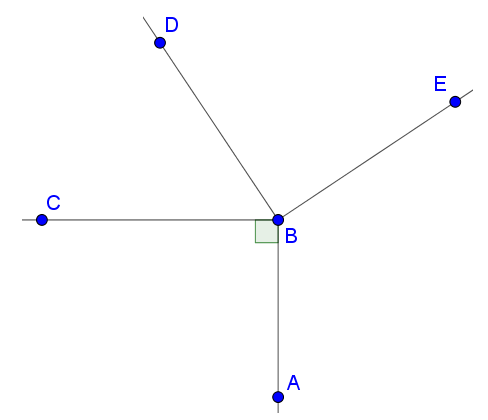1. Name an acute angle.
2. Name an obtuse angle.
3. Name a right angle.

### Problem 2

Points $Q$, $R$, and $T$ lie on a straight line, as shown below. Find the measure of $\angle SRT$.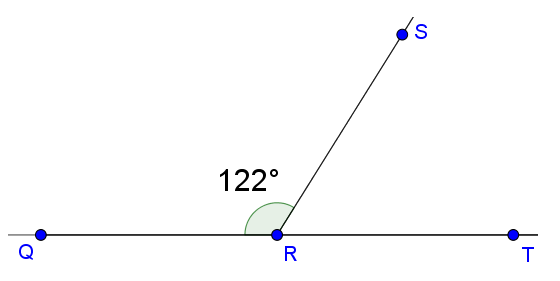Angle ${ABC}$ is a right angle. Find the value of $x$.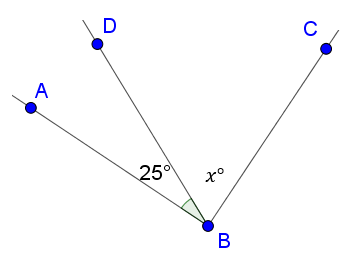### Problem 3

In the diagram below, point $P$ lies on line ${QT}$.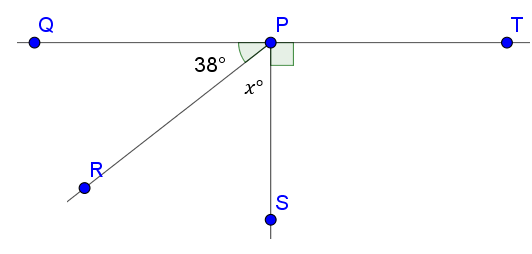1. Write and solve an equation to find the measure of $x$
2. What is the measure of $\angle RPT$?

## Problem Set

?The following resources include problems and activities aligned to the objective of the lesson that can be used to create your own problem set.

• Include problems where students practice identifying and determining angles in supplementary and complementary angle relationships.
• Include problems where students write simple equations to represent the relationship between a missing angle and its supplementary or complementary pair.

?

In the diagram below, point $A$ lies on line ${BD}$  and $\angle CAE$ is a right angle.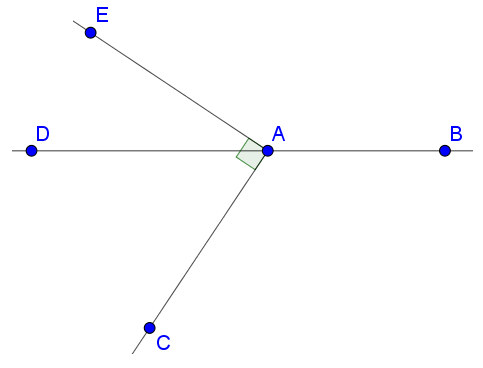1. Describe the relationship between $\angle DAE$ and $\angle EAB$.
2. Name two angles that are complementary.
3. If the measure of angle $DAC$ is 74°, what is the measure of angle $DAE$?

?